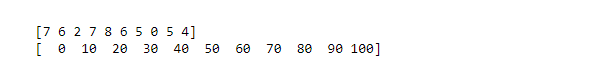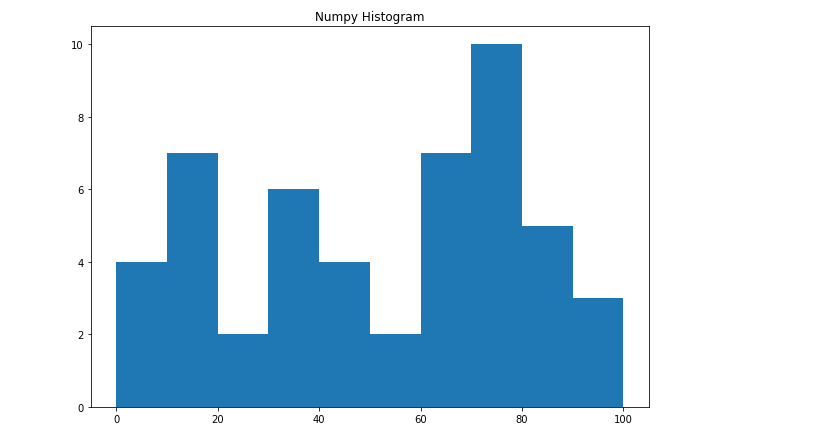Open in App
Not now

# NumPy.histogram() Method in Python

• Last Updated : 16 Dec, 2021

A histogram is the best way to visualize the frequency distribution of a dataset by splitting it into small equal-sized intervals called bins. The Numpy histogram function is similar to the hist() function of matplotlib library, the only difference is that the Numpy histogram gives the numerical representation of the dataset while the hist() gives graphical representation of the dataset.

## Creating Numpy Histogram

Numpy has a built-in numpy.histogram() function which represents the frequency of data distribution in the graphical form. The rectangles having equal horizontal size corresponds to class interval called bin and variable height corresponding to the frequency.
Syntax:

numpy.histogram(data, bins=10, range=None, normed=None, weights=None, density=None)

Attributes of the above function are listed below:

The function has two return values hist which gives the array of values of the histogram, and edge_bin which is an array of float datatype containing the bin edges having length one more than the hist.
Example:

## Python3

 `# Import libraries``import` `numpy as np`  `# Creating dataset``a ``=` `np.random.randint(``100``, size ``=``(``50``))` `# Creating histogram``np.histogram(a, bins ``=` `[``0``, ``10``, ``20``, ``30``, ``40``,``                        ``50``, ``60``, ``70``, ``80``, ``90``,``                        ``100``])` `hist, bins ``=` `np.histogram(a, bins ``=` `[``0``, ``10``,``                                     ``20``, ``30``,``                                     ``40``, ``50``,``                                     ``60``, ``70``,``                                     ``80``, ``90``,``                                     ``100``])` `# printing histogram``print``()``print` `(hist)``print` `(bins)``print``()`

Output:## Graphical representation

The above numeric representation of histogram can be converted into a graphical form.The plt() function present in pyplot submodule of Matplotlib takes the array of dataset and array of bin as parameter and creates a histogram of the corresponding data values.
Example:

## Python3

 `# import libraries``from` `matplotlib ``import` `pyplot as plt``import` `numpy as np `  `# Creating dataset``a ``=` `np.random.randint(``100``, size ``=``(``50``))` `# Creating plot``fig ``=` `plt.figure(figsize ``=``(``10``, ``7``))` `plt.hist(a, bins ``=` `[``0``, ``10``, ``20``, ``30``,``                    ``40``, ``50``, ``60``, ``70``,``                    ``80``, ``90``, ``100``])` `plt.title("Numpy Histogram")` `# show plot``plt.show()`

Output:My Personal Notes arrow_drop_up